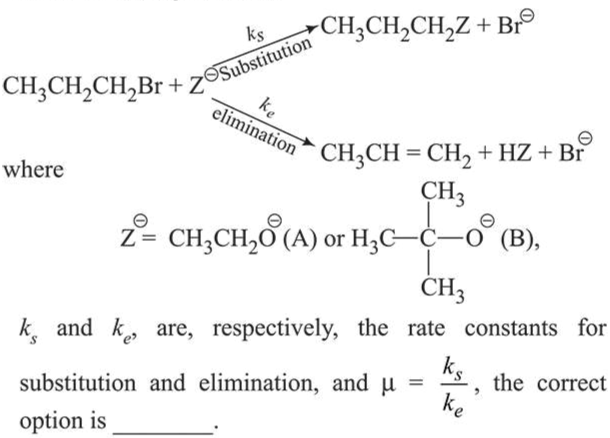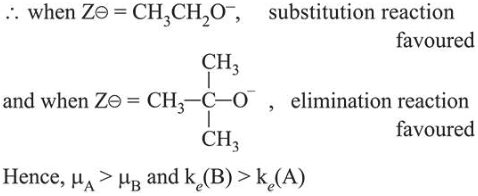# For the following reactionsQuestion:

For the following reactions1. $\mu_{\mathrm{B}}>\mu_{\mathrm{A}}$ and $k_{e}(\mathrm{~A})>k_{e}(\mathrm{~B})$

2. $\mu_{\mathrm{A}}>\mu_{\mathrm{B}}$ and $k_{e}(\mathrm{~B})>k_{e}(\mathrm{~A})$

3. $\mu_{\mathrm{B}}>\mu_{\mathrm{A}}$ and $k_{e}(\mathrm{~B})>k_{e}(\mathrm{~A})$

4. $\mu_{\mathrm{A}}>\mu_{\mathrm{B}}$ and $k_{e}(\mathrm{~A})>k_{e}(\mathrm{~B})$

Correct Option: , 2

Solution:

Among the given bases (A) and (B), $t$-butoxide being bulky base favours elimination reaction and ethoxide favours substitution reaction.